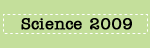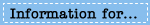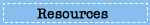# Playground Soil Response Page

In this 20-minute task, students investigate the permeability of soil samples from two sites a town is considering for a play area. Students use their results to help decide which site has the better water drainage and is therefore the better place for a grassy play area.

# Question 1

Based on the composition of soil sample A and soil sample B, which soil do you think will have greater permeability?

A. Soil A
B. Soil B

Explain why you think so in terms of the characteristics of the soils.

Sample Complete Student Response:

Soil A has better permeability because it consists of larger rocks, which concludes that it has more space in between for movement. That space will allow more water to flow throw, and thus will be more permeable.

#### Scoring Guide

Complete:
Student selects (A) Soil A or (B) Soil B, and provides a valid explanation supporting their selection. Explanation includes a soil characteristic that affects permeability (size, shape, connectivity of space between soil particles; size, shape, packing of soil particles), and relates the characteristic to the rate at which water flows through the soil. For example, more space between soil particles allows water to flow more quickly through the soil.

Partial:
Student response selects (A) or (B), and provides an explanation including a soil characteristic that affects permeability without adequately relating the characteristic to the rate at which water flows through the soil.

Unsatisfactory/Incorrect:
Student response is inadequate or incorrect.

Percentage of eighth-grade students in each response category: 2009
Complete Partial Unsatisfactory/Incorrect Omitted
29 21 48 1
NOTE: Detail may not sum to totals because of rounding.

# Question 2

Describe what data should be collected to calculate the permeability of each soil sample.

Explain how to use these data to calculate the permeability of each soil.

Sample Complete Student Response:

They should collect the number of water (in milliliters) that fell in to the container and how long it took for the water to get in the container. After you measured the water in the container by milliliters then you divide by the time which is 30 minutes.

#### Scoring Guide

Complete:
Student response indicates that the data to collect is the volume of water that has flowed through each soil into the beaker below (and the amount of time the water was allowed to flow through the soil), and explains that permeability is calculated by dividing the volume of water collected in each beaker by the time (30 minutes).

Partial:
Student response indicates that the data to collect is the volume of water that has flowed through each soil into the beaker below.

OR

Student response explains that permeability is calculated by dividing the volume of water by the time.

Unsatisfactory/Incorrect:
Student response is inadequate or incorrect.

Percentage of eighth-grade students in each response category: 2009
Complete Partial Unsatisfactory/Incorrect Omitted
7 64 26 2
NOTE: Detail may not sum to totals because of rounding.

# Questions 3 and 4

For each soil sample, record the volume of water that has flowed into each beaker.

Soil A: water volume (mL)

Soil B: water volume (mL)

Calculate the permeability for each soil sample in milliliters per minute. Round your answers to the nearest tenth.

Soil A: permeability (mL/min)

Soil B: permeability (mL/min)

Sample Complete Student Response:

90mL, 3.0mL/min, 10mL, 0.3mL/min

#### Scoring Guide

Part A: Volume of water in Soil A
Part B: Permeability of Soil A
Part C: Volume of water in Soil B
Part D: Permeability of Soil B

Part A

Complete:
Student response states correct water volume for Soil A in the range of 87 (mL) - 93 (mL). Units are not required, but response is not credited for an incorrect unit that refers to a non-volume unit of measure.

Unsatisfactory/Incorrect:
Student response is inadequate or incorrect.

Part B

Complete:
Student response states 3 (mL/min, 90/30). Units are not required, but response is not credited for an incorrect unit that refers to a non-rate unit of measure.

OR

Student response uses an incorrect volume stated in Part A, and performs the calculation correctly.

Unsatisfactory/Incorrect:
Student response is inadequate or incorrect.

Part C

Complete:
Student response states correct water volume for Soil B in the range of 7 (mL) -13 (mL). Units are not required, but response is not credited for an incorrect unit that refers to a non-volume unit of measure.

Unsatisfactory/Incorrect:
Student response is inadequate or incorrect.

Part D

Complete:
Student response states 0.3 (mL/min, 10/30). Units are not required, but response is not credited for an incorrect unit that refers to a non-rate unit of measure.

OR

Student response uses an incorrect volume stated in Part C, and performs the calculation correctly.

Unsatisfactory/Incorrect:
Student response is inadequate or incorrect.

Composite Score:
Student response received one of four possible composite scores (Complete, Essential, Partial, Unsatisfactory/Incorrect) based on the student's combined performance on Parts A, B, C, and D of the item. For example, a student response Complete for Part A, Complete for Part B, Complete for Part C, and Unsatisfactory/Incorrect for Part D received a composite score of Essential.

Composite Score Part A Part B Part C Part D

Complete

Complete Complete Complete Complete

Essential

Unsatisfactory/Incorrect Complete Complete Complete
Complete Unsatisfactory/Incorrect Complete Complete
Complete Complete Unsatisfactory/Incorrect Complete
Complete Complete Complete Unsatisfactory/Incorrect

Partial

Complete Complete Unsatisfactory/Incorrect Unsatisfactory/Incorrect
Complete Unsatisfactory/Incorrect Unsatisfactory/Incorrect Complete
Unsatisfactory/Incorrect Unsatisfactory/Incorrect Complete Complete
Complete Unsatisfactory/Incorrect Complete Unsatisfactory/Incorrect
Unsatisfactory/Incorrect Complete Unsatisfactory/Incorrect Complete
Complete Unsatisfactory/Incorrect Unsatisfactory/Incorrect Unsatisfactory/Incorrect
Unsatisfactory/Incorrect Complete Unsatisfactory/Incorrect Unsatisfactory/Incorrect
Unsatisfactory/Incorrect Unsatisfactory/Incorrect Complete Unsatisfactory/Incorrect
Unsatisfactory/Incorrect Unsatisfactory/Incorrect Unsatisfactory/Incorrect Complete
Unsatisfactory/Incorrect Unsatisfactory/Incorrect Unsatisfactory/Incorrect Unsatisfactory/Incorrect Unsatisfactory/Incorrect

Percentage of eighth-grade students in each response category: 2009
Complete Essential Partial Unsatisfactory/Incorrect Omitted
17 14 60 9 #
# Rounds to zero.
NOTE: Detail may not sum to totals because of rounding.

# Question 5

The soil of the site for the play area needs to have good water drainage so that it does not become too wet and muddy to use at any time of the year.

Another student repeated the experiments you just ran to calculate the permeabilities of the two soils.

The student’s results are shown below.

Soil A at Site A

Permeability: 3.0 mL/min
Soil B at Site B
Permeability: 0.3 mL/min

Based on these data, which soil would be best to use for the play area?

A. Soil A
B. Soil B

Explain how you know. Use the data to support your explanation.

Sample Complete Student Response:

The soil in site A is more permeable, meaning the water goes quicker through the soil resulting in faster drainage. The permeability in site A is 2.7 mL/min more than Site B, therefore it is better for the playground.

#### Scoring Guide

Complete:
Student response selects Soil A, and provides a correct explanation that relates the higher permeability results (faster flow rate) of Soil A to better drainage (less muddiness) at Site A.

Partial:
Student response selects Soil A, and provides an explanation that refers to the higher permeability results of Soil A.

OR

Student response selects Soil A, and provides an explanation that refers to the better drainage (less muddiness) at Site A.

Unsatisfactory/Incorrect:
Student response is inadequate or incorrect.

Percentage of eighth-grade students in each response category: 2009
Complete Partial Unsatisfactory/Incorrect Omitted
11 41 48 #
# Rounds to zero.
NOTE: Detail may not sum to totals because of rounding.

SOURCE: U.S. Department of Education, Institute of Education Sciences, National Center for Education Statistics, National Assessment of Educational Progress (NAEP), 2009 Science Assessment.

•••